Clock's gears

In the clock machine, three gears fit together. The largest has 168 teeth, the middle 90 teeth, and the smallest 48 teeth. The middle wheel turns around its axis in 90 seconds. How many times during the day do all the gears meet in the starting position?

Result

n =  17

Solution:Leave us a comment of this math problem and its solution (i.e. if it is still somewhat unclear...):Be the first to comment!To solve this verbal math problem are needed these knowledge from mathematics:

Do you want to calculate least common multiple two or more numbers?

Next similar math problems:

1. Game roomWinner can took win in three types of jettons with value 3, 30 and 100 dollars. What is minimal value of win payable in this values of jettons?
2. 3 buses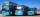At morning 5:00 am three buses started from one place. The first travel in five-minute intervals, the second at 10-minute intervals and the third at 25-minute intervals. At what hour will again be the three buses coming from the same place?
3. BiketrialKamil was biketrial. Before hill he set the forward gear with 42 teeth and the back with 35 teeth. After how many exercises (rotation) of the front wheel both wheels reach the same position?
4. LCM of two numberFind the smallest multiple of 63 and 147
5. Lcm 2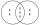Create the smallest possible number that is divisible by numbers 5,8,9,4,3
6. Dance ensemble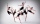The dance ensemble took the stage in pairs. During dancing, the dancers gradually formed groups of four, six and nine. How many dancers have an ensemble?
7. Six-digit primesFind all six-digit prime numbers that contain each one of digits 1,2,4,5,7 and 8 just once. How many are they?
8. Apples 2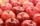How many minimum apples are in the cart, if possible is completely divided into packages of 6, 14 and 21 apples?
9. Trams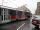Trams have an average speed 23 km/h and run in tact 14 minutes. Pedestrian walking speed is 3.3 km/h. At what intervals trams outrun pedestrian?
10. Primes 2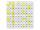Which prime numbers is number 2025 divisible?
11. Cyclist vs car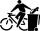Cyclist rode out of the city at 18 km/h. 1 hour 30 minutes behind him started car and caught up with the cyclist in 50 minutes. How fast was driving the car? Where (what kilometer) from the city car overtook a cyclist?
12. Dance groupThe dance group formed groups of 4, 5, and 6 members. Always one dancer remains. How many dancers were there in the whole group?
13. MO C–I–1 2018An unknown number is divisible by just four numbers from the set {6, 15, 20, 21, 70}. Determine which ones.
14. Reminder and quotientThere are given the number C = 281, D = 201. Find the highest natural number S so that the C:S, D:S are with the remainder of 1,
15. The florist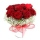The florist had 200 roses in the morning. During the day, more than half sold it. From the remaining roses, it will tie the bouquet. If a bouquet of 3, 4, 5 or 6 roses are bound, one always remains. How many roses from the morning shipment sold?
16. PlumsIn the bowl are plums. How many would be there if we can divide it equally among 8, 10 and 11 children?
17. Digit sumDetermine for how many integers greater than 900 and less than 1,001 has digit sum digit of the digit sum number 1.